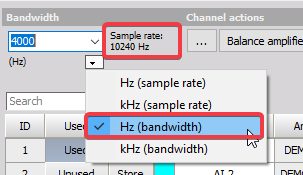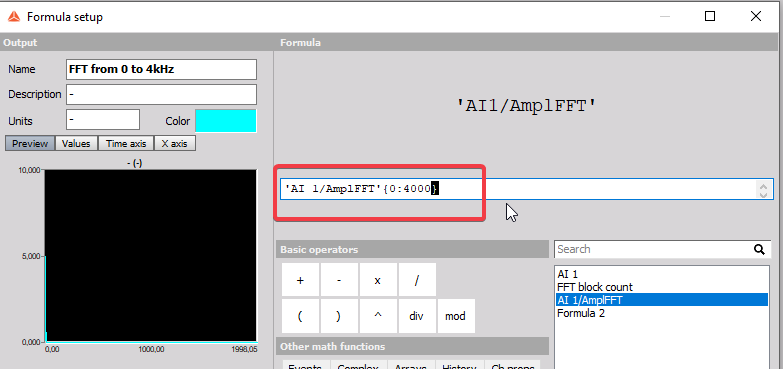EN

English
French
German
Italian
Russian
Languages

# Specifying FFT Frequency Range

Alexandre Young

Posted on 15.10.2019 08:02

Hello,

I am looking for a method to perform an FFT calculation over a specified frequency range, say 0-4kHz. However, my data sampling rate was set to 20kHz, thus the FFT is calculated for 0-10kHz by default.

From reading the Dewesoft manual, I do not see any obvious way to get around this :

"The maximum frequency of the FFT is half of the signal sampling frequency"

My understanding of FFT is, that the vibration amplitudes calculated are dependent on the total frequency range of the FFT. Is this correct? Alternatively, if FFT amplitude is dependent only on the number of analysis lines used, could I alter this to match the required frequency resolution in the desired range (0-4kHz).

Jernej Sirk
DSA Application Engineer
Posted on 16.10.2019 08:32

Dear Mr. Young,

the easiest way to define the FFT in a range from 0 to 4kHz is to define bandwidth insted of sample rate in Analog in.

When defining the bandwidth, sample rate will be calculated accordingly.If you are using bandwidth up to 10kHz and want to Calculate FFT only up to 4kHz, the FFT boundaries can be defined in a Formula.

The input is the output channel from FFT math and with {} you define the wanted range.Best regards,

Jernej Sirk